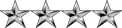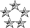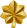## How to Solve Questions like these? BIT Practice Quiz

Mathematics for Computing
Nipuna
ModeratorPosts: 2729
Joined: Mon Jan 04, 2010 8:02 pm
Location: Deraniyagala,SRI LANKA

### How to Solve Questions like these? BIT Practice Quiz

These are from BIT Practice Quiz.

But I Don't know how to solve them.

Can any one Explain them?Q1.jpg (57.73 KiB) Viewed 5370 timesQ2.jpg (47.39 KiB) Viewed 5370 timesQ3.jpg (31.93 KiB) Viewed 5370 timesQ4.jpg (56.04 KiB) Viewed 5370 times
I am Adding this in BIT forum Too.
NeoPosts: 2642
Joined: Wed Jul 15, 2009 2:07 am
Location: Colombo

### Re: How to Solve Questions like these? BIT Practice Quiz

I am Adding this in BIT forum Too.
Did you get an answer. I don't think so.
Just because, you need to do the exercises yourself. Otherwise you will never learn.
By getting someone to do it for you will give you a definite 'F' for the subjectHowever to give you a start I'll answer the questions (BTW: my memory is not good on these).

You can always ask questions like what is bijection. What is g ∘ f means.. things like that. But not exercises.

First read Function composition. Very nice article from wiki.
g ∘ f is read as g circle f and it does the operation g( f(x) ).

7. I don't see the question here.
However I see something very easy here.
f5 ∘ f4 ∘ f3 ∘ f2 ∘ f1 = +

since for all x in A,
f5(f4(f3(f2(f1(x))))) = +

f1 and f4 are not onto functions (Also known as non-surjective function). In f1, there is 3 in B and in f4, there is x, y.

f2 and f5 are both one-to-one and onto functions (Also known as bijective function).

f3 is an onto function (Also known as Surjective function) but NOT one-to-one.

8.
A is correct
B is correct
C is not correct. Reason: This is not always true since Function composition is not not always commutative.

FYI:
The functions g and f are said to commute with each other if g ∘ f = f ∘ g. In general, composition of functions will not be commutative. Commutativity is a special property, attained only by particular functions, and often in special circumstances. But a function always commutes with its inverse to produce the identity mapping.

In simple terms, if there is a function f defined as f(x) = x. Then f-1(x)=x. Such a function is called an identity function. In that case f-1 ∘ f = f ∘ f-1. The identity function f on M is often denoted by idM.

However, the composition of functions is always associative. That is, if f, g, and h are three functions with suitably chosen domains and codomains, then f ∘ (g ∘ h) = (f ∘ g) ∘ h.

11.
(b) and (d) are correct.

f(x) = x2 + 1
g(x) = 3x - 2

f(g(x)) = f ∘ g = f(3x - 2)
= (3x - 2)2 + 1
= (9x2 - 12x + 4) + 1
= 9x2 - 12x + 5

g(f(x)) = g ∘ f = g(x2 + 1)
= 3(x2 + 1) - 2
= 3x2 + 3 - 2
= 3x2 + 1

I'll answer (12) later... I need to refresh my mind with relations on pairs.
Nipuna
ModeratorPosts: 2729
Joined: Mon Jan 04, 2010 8:02 pm
Location: Deraniyagala,SRI LANKA

### Re: How to Solve Questions like these? BIT Practice Quiz

Thanks a Lot Friend.

And One Student Replied Me. She is " HC JAYASINGHE " I must Grateful to her too as you. And She Helped me with Question 7.

I am Going to study these.

By The Way, The Problem I couldn't Study these in BIT VLE is BIT VLE only has Topics but they only Explained Relations. I think they missed to add About Functions. That's Why I was confused.
NeoPosts: 2642
Joined: Wed Jul 15, 2009 2:07 am
Location: Colombo

### Re: How to Solve Questions like these? BIT Practice Quiz

12.
(a) and (b) are wrong. (c) is correct.
I'm not sure the last two. I guess only (e) is correct out of them. Refer to the definition.
Herath
MajorPosts: 417
Joined: Thu Aug 05, 2010 7:09 pm

### Re: How to Solve Questions like these? BIT Practice Quiz

Neo's answers are perfect. These are from Calculus and Introductory Real Analysis. Don't get upset if you can not understand them at the first try. Sometimes they are confusing unless you don't break it down to simple parts and solve.Nipuna
ModeratorPosts: 2729
Joined: Mon Jan 04, 2010 8:02 pm
Location: Deraniyagala,SRI LANKA

### Re: How to Solve Questions like these? BIT Practice Quiz

Thanks Friend Herath. After a Long Time.